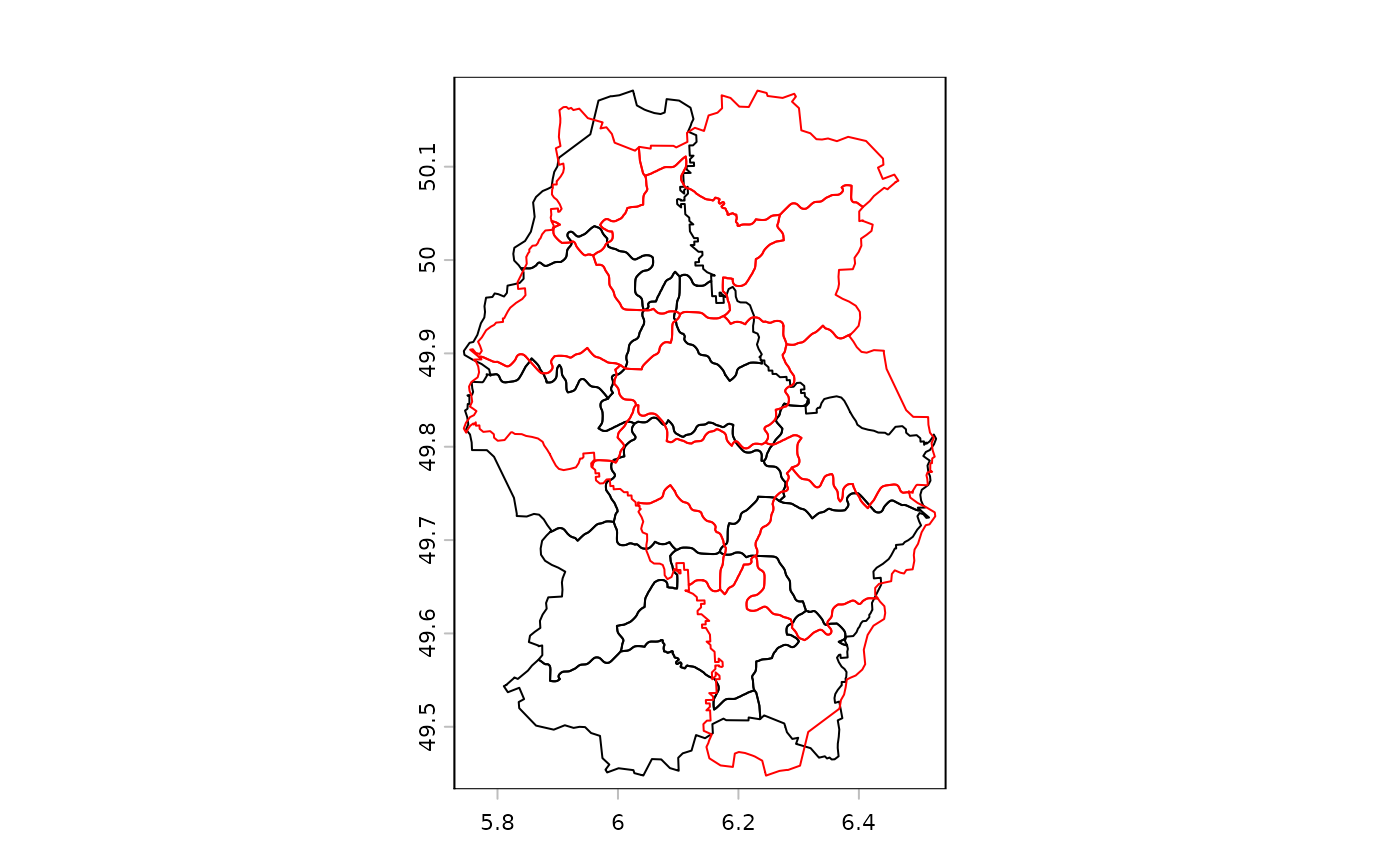Spin (rotate) the geometry of a SpatVector.

# S4 method for SpatVector
spin(x, angle, x0, y0)

## Arguments

x

SpatVector

angle

numeric. Angle of rotation in degrees

x0

numeric. x-coordinate of the center of rotation. If missing, the center of the extent of x is used

y0

numeric. y-coordinate of the center of rotation. If missing, the center of the extent of x is used

## Value

SpatVector

rescale, t, shift

## Examples

f <- system.file("ex/lux.shp", package="terra")
v <- vect(f)
w <- spin(v, 180)
plot(v)
lines(w, col="red")# lower-right corner as center
e <- as.vector(ext(v))
x <- spin(v, 45, e, e)